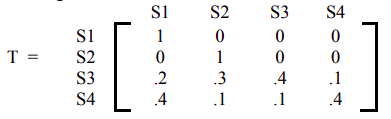# 10.5: CHAPTER REVIEW

$$\newcommand{\vecs}{\overset { \rightharpoonup} {\mathbf{#1}} }$$ $$\newcommand{\vecd}{\overset{-\!-\!\rightharpoonup}{\vphantom{a}\smash {#1}}}$$$$\newcommand{\id}{\mathrm{id}}$$ $$\newcommand{\Span}{\mathrm{span}}$$ $$\newcommand{\kernel}{\mathrm{null}\,}$$ $$\newcommand{\range}{\mathrm{range}\,}$$ $$\newcommand{\RealPart}{\mathrm{Re}}$$ $$\newcommand{\ImaginaryPart}{\mathrm{Im}}$$ $$\newcommand{\Argument}{\mathrm{Arg}}$$ $$\newcommand{\norm}{\| #1 \|}$$ $$\newcommand{\inner}{\langle #1, #2 \rangle}$$ $$\newcommand{\Span}{\mathrm{span}}$$ $$\newcommand{\id}{\mathrm{id}}$$ $$\newcommand{\Span}{\mathrm{span}}$$ $$\newcommand{\kernel}{\mathrm{null}\,}$$ $$\newcommand{\range}{\mathrm{range}\,}$$ $$\newcommand{\RealPart}{\mathrm{Re}}$$ $$\newcommand{\ImaginaryPart}{\mathrm{Im}}$$ $$\newcommand{\Argument}{\mathrm{Arg}}$$ $$\newcommand{\norm}{\| #1 \|}$$ $$\newcommand{\inner}{\langle #1, #2 \rangle}$$ $$\newcommand{\Span}{\mathrm{span}}$$$$\newcommand{\AA}{\unicode[.8,0]{x212B}}$$

## SECTION 10.5 PROBLEM SET: CHAPTER REVIEW

1. Is the matrix given below a transition matrix for a Markov chain? Explain.
1. $$\left[\begin{array}{ccc} .1 & .4 & .5 \\ .5 & -.3 & .8 \\ .3 & .4 & .3 \end{array}\right]$$
2. $$\left[\begin{array}{ccc} .2 & .6 & .2 \\ 0 & 0 & 0 \\ .3 & .4 & .5 \end{array}\right]$$
2. A survey of computer buyers indicates that if a person buys an Apple computer, there is an 80% chance that their next purchase will be an Apple, while owners of a Windows computer will buy an Windows computer again with a probability of .70. The buying habits of these consumers are represented in the transition matrix below.1. Find the probability that a present owner of an Apple computer will buy a Windows computer as his next computer.
2. Find the probability that a present owner of an Apple computer will buy a Windows computer as his third computer.
3. Find the probability that a present owner of a Windows computer will buy a Windows computer as his fourth computer.
1. Professor Trayer either teaches Finite Math or Statistics each quarter. She never teaches Finite Math two consecutive quarters, but if she teaches Statistics one quarter, then the next quarter she will teach Statistics with a 1/3 probability.
1. Write a transition matrix for this problem.
2. If Professor Trayer teaches Finite Math in the Fall quarter, what is the probability that she will teach Statistics in the Winter quarter.
3. If Professor Trayer teaches Finite Math in the Fall quarter, what is the probability that she will teach Statistics in the Spring quarter.
2. Determine whether the following matrices are regular Markov chains.
1. $$\left[\begin{array}{ll} 1 & 0 \\ .3 & .7 \end{array}\right]$$
2. $$\left[\begin{array}{lll} .2 & .4 & .4 \\ .6 & .4 & 0 \\ .3 & .2 & .5 \end{array}\right]$$
3. The transition matrix for switching academic majors each quarter by students at a university is given below, where Science, Business, and Liberal Arts majors are denoted by S, B, and A, respectively.1. Find the probability of a science major switching to a business major during their first quarter.
2. Find the probability of a business major switching to a Liberal Arts during their second quarter.
3. Find the probability of a science major switching to Liberal Artsr during their third quarter.
1. John Elway, the football quarterback for the Denver Broncos, called his own plays. At every play he had to decide to either pass the ball or hand it off. The transition matrix for his plays is given in the following table, where P represents a pass and H a handoff.1. If John Elway threw a pass on the initial play, what is the probability that he handed handoff on the two plays later?
2. Determine the long term play distribution.
1. Company I, Company II, and Company III compete against each other, and the transition matrix for people switching from company to company each year is given below.1. If the initial market share is 20% for Company I, 30% for Company II and 50% for Company III, what will the market share be after the next year?
2. If this trend continues, what is the long range expectation for the market?
1. Given the following absorbing Markov chain.1. Identify the absorbing states.
2. Write the solution matrix.
3. Starting from state 4, what is the probability of eventual absorption in state 1?
4. Starting from state 3, what is the probability of eventual absorption in state 2?
1. A mouse placed in the maze moves from room to room randomly. From any room, the mouse will choose a door to the next room with equal probabilities. Once it reaches room 1, it finds food and never leaves that room. And when it reaches room 6, it is trapped and cannot leave that room. What is the probability that the mouse will end up in room 1 if it was initially placed in room 3?1. What is the probability that the mouse will end up in room 6 if it was initially in room 2?

This page titled 10.5: CHAPTER REVIEW is shared under a CC BY 4.0 license and was authored, remixed, and/or curated by Rupinder Sekhon and Roberta Bloom via source content that was edited to the style and standards of the LibreTexts platform; a detailed edit history is available upon request.